﻿ Mapping Chromosome Sequences of Several Primate on Variant Maps

# Journal of Computer Science & Systems BiologyOpen Access

Like us on:

• Research Article
• J Comput Sci Syst Biol 2019, Vol 12(2): 297
• DOI: 10.4172/0974-7230.1000297

# Mapping Chromosome Sequences of Several Primate on Variant Maps

Huaxian Zheng* and Jeffrey Zheng
School of Software, Yunnan University, Kunming, China
*Corresponding Author: Huaxian Zheng, School of Software, Yunnan University, Kunming, China, Tel: +8618388308955, Email: [email protected]

Received Date: Apr 03, 2019 / Accepted Date: Apr 15, 2019 / Published Date: Apr 22, 2019

### Abstract

The chromosome is a carrier of genetic information. The number of somatic chromosomes in normal people is 23 pairs, and there have shapes and structures. It has been found that there are more than 100 kinds of chromosomal diseases caused by chromosomal abnormalities. Chromosome diseases can often cause miscarriage, congenital, congenital multiple malformations, cancer, etc. At present the research on chromosomal sequences has carried out. People have been looking for a suitable visualization model. In this type of visualization models, there is no problem of information degradation and data loss, and a complete chromosomal sequence distribution feature can be mapped. There are multiple sets of chromosomal sequences in species, and a comparative analysis is needed to find out some of the relationships between chromosomes in humans during evolution. In this paper, variant maps are used to illustrate the segmentation probability of the chromosome sequences of Homo sapiens and non-human primate species, distributions of different chromosomal sequence features are compared and analyzed by multiple two-dimension statistical probability maps.

Keywords: Chromosomal sequence; Probability measurement; Twodimension statistics; Probability maps

#### Introduction

Using advanced sequencing technologies, human, chimpanzee, gorilla and other primates and non-primate genomes are gradually sequenced, it is possible to analyze the relationship between human and other primates and non-primate chromosomal sequences from a genomic perspective. During the evolution of primates, some species produced specific chromosomal rearrangements. Almost all the scorpion animals have 48 chromosomes, while chimpanzees, gorillas, and orangutans have no exception. Only human alone have 46 chromosomes [1,2]. The study found that human has two chromosomes connected end to end to form chromosome 2 in the process of evolution. In Figure 1, the genes on chromosome 2 of Homo sapiens can be compared with 12 (or 2A) and 13 of scorpions. Or the genes on chromosome (2B) correspond one-to-one.

Figure 1 shows that the Synchromos in SYNTENY PORTAL  compares the entire chromosomal gene distribution of Homo sapiens with the chimpanzee’s whole-chromosomal gene distribution. (a) shows the relationship between Homo sapiens and chimpanzees on the whole chromosome. (b) indicates that the Homo sapiens chromosome 2 gene sequence is distributed on two chromosomes of chimpanzees, 2A and 2B.

With the rapid development of gene sequencing technology, the amount of data in gene databases is huge, and the comparison algorithm is limited by time complexity when performing whole genomes, so non-contrast sequence analysis methods have emerged. The use of graphs or numerical values to represent biological sequences, and the relationship between biological sequences or the values, and thus the relationship of biological sequences is one of the non-contrast analytical methods. Mapping a biological sequence to a graph through a mapping relationship can handle a large number of gene sequences, and the numerical representation of the biological sequence is mainly mathematical methods. Variant maps make it easier to analyze the gene sequence. Different from traditional graphics, this paper is focused on presenting the hidden information and rules in the data through visualization. Two-dimensional visualization of chromosome sequences based on probability theory statistical methods reveal the relationship between each base in the chromosome sequence as variant maps. The results of visualization of chromosome sequences under different measurement conditions are also listed.

At present, there are many visualization models of biological sequences, and they mainly work on DNA sequences. Although many visualization models have achieved good results , they still have their defects, such as DNA sequence spectral type two-dimensional visualization model by DNA sequences. Transforming into a two-dimensional curve enables visualization of DNA sequences. However, this model can reflect the nature of some DNA sequences for shorter DNA sequences, but this model is not suitable for visualization of long DNA sequences . The DNA vector double vector two-dimensional visualization model is also a more common DNA sequence visualization model. It uses DNA walking technology to encode four bases into motion vectors in two directions. However, as the length of the DNA sequence increase, the whole curve is only a trend, which will cause some details lost. Therefore, it may cause the human eye to ignore some important information. In this paper, the variable probability statistical visualization method is proposed. Firstly, the current status of DNA sequence visualization method is analyzed. Secondly, the model for processing the whole framework and measurement sequence of chromosome gene sequence is introduced. Finally, the above measurement model is used to visualize the chromosome sequence. The illustration shows the analysis. The contributions of this article are as follows:

(1) The distribution of bases in an entire chromosome sequence can be displayed;

(2) The original chromosomal sequence is numerically quantified by probability, and some corresponding relationships between the bases are shown in the illustrated results;

(3) The difference in distribution between each chromosome can be easily observed in the graphical results.

#### Module and Methods

The overall framework structure for processing chromosomal gene sequences is shown in Figure 2.

Measuring module

1. S=s1, s2, … si…sn, si∈{A, G, C, T};

2. {A, G, T, C}=D, V ∈ D;

3. Processing the S sequence into the 01 sequence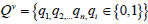;

4. Segment Q according to the length of the sequence, and the segment sequence is represented as a segmen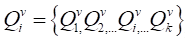;

5. Statistical quantity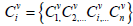;

6. Non-normalized probability measure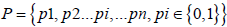;

7. Normalized probability measure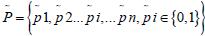;

8. Statistics of the number of non-normalized probabilities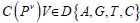;

9. Statistical normalized probability quantity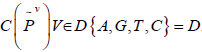.

Projection module

In this paper, the thermogram principle is used to realize the diagram. When the non-normalized graph is used, the probability measure C(PV) is taken as the horizontal and vertical coordinates, and the non-normalized graph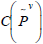is used as the horizontal and vertical coordinates.

Measurement methods

From the original chromosomal gene sequence data to the final two-dimension feature distribution map, the following steps are mainly taken: data classification, data processing, and data visualization.

The genome-wide sequence data of primate species contains autosomes and sex chromosomes. In the data processing, one chromosome sequence is directly processed. Each chromatid can be regarded as a double-helix DNA molecule, and deoxynucleotides It is composed of deoxyribose, phosphoric acid and nitrogenous bases. There are four bases, adenine (A), guanine (G), cytosine (C) and thymine (T), so gene sequence is regarded as a string of four characters A, G, C, and T, which can be expressed as:S=s1s2…si…sn,si∈{A,G,C,T}, The sequence length is denoted by N, and the sequence is divided into k subsequence segments by length m. The number of A (adenine), G (guanine), C (cytosine), and T (thymine) in each subsequence is counted, and the corresponding number is represented by CA, CG, CC, and CT, respectively.

Based on the measurement parameters indicated by N, the statistics corresponding to the probability measure are performed. The probability measures are divided into two types, normalized percentage and non-normalized percentage . Non-normalized measure: the number of bases divided by the length of the subsequence to obtain a percentage; normalization measure: the number of bases divided by the number of two complementary bases. The normalized measure of each base corresponds to a percentage between 0 and 1. The data is used as the input of the coordinate position mapping part, and processed according to certain rules, and finally the horizontal and vertical of each point are obtained. Coordinate .

Visualization Results

In this paper, the numerical values of the probability and statistics are visualized by using the principle of heat map, which indicates the intensity of points in the form of special brightness. In the figure, Z represents the color change corresponding to the intensity of the dots, and the number is from low to high, the color is dark to special highlight. The central part of the graph obtained in this paper is the area with the densest point distribution, and the intensity of points gradually decreases with the edge diffusion.

Using the primate chromosomal sequences, the two-dimensional feature distribution map is formed by controlling the controllable parameters, and the characteristic distribution map is obtained by controlling the variables of the horizontal, vertical coordinates and the segment length m, feature3 is shown below. The different nature of the base is divided on three levels according to the four bases :

Purine R=A, G/ Pyrimidine Y=C, T

Amino M=A, C/Carbonyl K=G, T

Strong hydrogen bond S=G, C/weak hydrogen bond W=A, T;

Figure 3 (a1) (b1) is obtained by the probability of purine A and the probability of G;

Figure 3 (a2) (b2) with the probability of pyrimidine C and the probability of T;

Figure 3 (a3) (b3) with the probability of amino A and the probability of C;

Figure 3 (a4) (b4) with the probability of carbonyl G and the probability of T;

Figure 3 (a5) (b5) with the probability of strong hydrogen bond G and the probability of C;

Figure 3 (a6) (b6) with the probability of weak hydrogen bond A and the probability of T are obtained in.

Figure 3 (Select sample is the chromosome 2 sequence of Homo sapiens).

Non-normalized feature map: (a1, a2, a3, a4, a5, a6); Normalized feature map: (b1, b2, b3, b4, b5, b6). Absc. X: A (adenine), C (cytosine), A (6-aminopurine), G (4-amino-2-carbonylpyrimidine), G, A; Ordinate Y: G (guanine), T (thymine), C (2-amino-6-hydroxyindole), T (5-methyluracil), C, T; The horizontal and vertical coordinates are in one-to-one correspondence to form a two-dimensional feature distribution map.

From the comparison of non-normalization and normalization in Figure 3, it will be found that the feature distribution points of (a1) and (a2) in the non-normalization, relative to (a3) or (a4) or (a5) or (a6) are more similar, and the feature distribution points of (a3) and (a4) are more similar, compared to the feature points of (a1) or (a2) or (a5) or (a6). This shows a distribution relationship between the bases. After normalization, the feature distribution points of (b1), (b2), (b3), and (b4) have certain commonalities. By rotating the maps, it is found that the four feature distribution maps are similar; In the normalized to normalized feature distribution map, we find that from (a5) to (b5), (a6) to (b6), the feature distribution changes greatly, and the horizontal and vertical coordinates of (a5) is the feature distribution map of G, C (a6). The horizontal and vertical coordinates of the feature distribution map correspond to the probability of A and T bases. It can be seen that the feature distribution map of (a5) and (a6) is symmetrically distributed. We know that GC, AT and their relationship is the base complementary pairing. From the result graph of (a5) (a6), the information we can obtain is that if there is a certain region in a whole chromosome sequence where the probability of distribution corresponding to one base and its complementary base (g, f), the probability distribution of the base and its complementary base must exist in another region of the chromosome (f, g), and the same reason, (b5) (b6) can be obtained after the normalization process, the characteristic distribution map shows the result which shows that the base and its complementary base from the feature distribution map of (b5)(b6) is the correlation rate distribution, the information we can get in the obtained graphical results is that when a base has a high probability of distribution in a certain region in a chromosomal sequence, the number of complementary base is relatively small, distributed in the region.

The amount of information that transmitted by an image can be represented by the information entropy of the image. The size of the information entropy basically reflects the texture transformation of each module. The information entropy is larger, the texture information is richer, and the selected feature points are correspondingly more.

Otherwise, the texture information does not change much, and the number of feature points selected is less . In this paper, by calculating the information entropy of each group of images, Table 1 calculates that the information entropy of the a1-b1 and a2-b2 groups is higher, so the variables of the a1-b1 group metric are selected as the variables of other chromosome sequences in the following.

Entropy value/Base combination Non-normalized entropy (Nne) Normalized entropy (Ne) Mean value of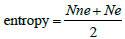human_2_AG 2.51903968594 2.18817945191 ˜2.35
human_2_CT 2.46934409505 2.18269916725 ˜2.33
human_2_AC 2.37927028283 2.18326183893 ˜2.28
human_2_GT 2.38912981063 2.18785821844 ˜2.29
human_2_GC 2.45460500987 0.85512410955 ˜1.65
human_2_AT 2.84052017053 0.879727950085 ˜1.86

Table 1: Image entropy table. The table shows the entropy values of the non-normalized and normalized maps of the Homo sapiens chromosome 2 sequence when the horizontal and vertical coordinates are different.

Firstly, this paper selects several relatively special chromosome sequences of Homo sapiens chromosome 2 and gorillas (the chromosome 2 sequence of Homo sapiens and the corresponding chromosomal sequence of gorillas is 2A, 2B), and chromosome 16 and chromosome 21 sequence abnormalities lead to humans For chromosomal diseases , the gorilla’s chromosome sequences 2A, 2B, 16, and 21 and their X chromosome sequences are selected for visualization of non-normalization and normalization (Figures 4 and 5).

Figure 4: Non-normalized distribution of chromosomes of chromosome 2 and gorillas of Homo sapiens.

Figure 5: Normalized distribution of chromosomes of chromosome 2 and gorillas of Homo sapiens.

The X chromosome is a sex determining genome of the organism of chromosome in the XY, Examples of mutations on the X chromosome include more common diseases such as color blindness, hemophilia, and fragile-X syndrome. Studies have shown that chimpanzees are the closest primate species to humans. From Figure 6a and 6b, the characteristic distribution maps are similar. It also proves that the probabilistic method used in this paper is effective and does not violate the original chromosomal sequence. In Figure 6, the corresponding distribution characteristics of the primate neutral chromosomes will also be found in Figure 7.

#### Conclusion

In this paper, a whole sequence of chromosomal sequences was sequenced based on the variable value representation method of probability and statistics, and the chromosomal sequences of several prominent primates were visualized, and the results were compared and analyzed. Some features of previous visualization models that were unable to observe the full base distribution due to the long sequence were implemented. The advantage of the visualization model in this paper is that the observer can observe the probability distribution between the bases of a whole chromosomal sequence that has been sequenced at present, and the graphical results also show the corresponding distribution relationship between the bases. When comparing the maps of Homo sapiens chromosomes with other primate chromosome sequences, it is easier to observe that there is a certain X eno-existence in the probability distribution between them, and the image distribution of species close to the Homo sapiens is more similar. Thus, a comparative analysis of the XOR relationship between each chromosome sequence is performed. The insufficiency of the visualization method in this paper is that the specific distribution position of the differential gene sequence cannot be determined when there is a difference in the distribution of the chromosomal sequence; in the illustrated results, it is shown that a base in a chromosomal sequence is related to its complementary base distribution. but it is not certain that there is a correlation between the base and its complementary base in the same region. This paper does not analyze the graphical results of abnormal chromosomal sequences and normal chromosomal sequences and needs further study. It is hoped that the visualization method given in the paper, the proposed analytical measurement model and the corresponding probability distribution characteristics between the chromosomal sequence bases shown in the figure can be the whole genome chromosomal sequence data of different species. And application research of structural visual analysis provides a solid model and practical foundation.

#### Acknowledgements

Thanks to the school of software Yunnan University, to the key laboratory of Yunnan software engineering for excellent working environment. Financial supports to this project are provided by National Science Foundation of China NSFC (No. K1020720) and the Overseas Higher-level Scholar Project of Yunnan Province, China (No. W8110305) and the Science and technology plan project of Yunnan Province, China (No. KC1810123).

#### References

Citation: Huaxian Zheng, Jeffrey Zheng (2019) Mapping Chromosome Sequences of Several Primate on Variant Maps. J Comput Sci Syst Biol 12:35-41. DOI: 10.4172/0974-7230.1000297

Copyright: © 2019 Zheng H, et al. This is an open-access article distributed under the terms of the Creative Commons Attribution License, which permits unrestricted use, distribution, and reproduction in any medium, provided the original author and source are credited.

Select your language of interest to view the total content in your interested language

##### Recommended Journals
Viewmore
• ###### 16th International Conference on Structural and Molecular Biology

January 23-24 ,2020 Bangkok, Thailand

Viewmore
###### Article Usage
• Total views: 772
• [From(publication date): 0-0 - Dec 07, 2019]
• Breakdown by view type
• HTML page views: 714Can't read the image? click here to refresh